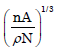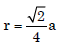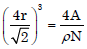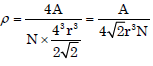Courses

# Basic Concepts (Structure Of Solids), Hardness Test & Crystalline Material - MCQ Test 1

## 20 Questions MCQ Test GATE Mechanical (ME) 2022 Mock Test Series | Basic Concepts (Structure Of Solids), Hardness Test & Crystalline Material - MCQ Test 1

Description
This mock test of Basic Concepts (Structure Of Solids), Hardness Test & Crystalline Material - MCQ Test 1 for Mechanical Engineering helps you for every Mechanical Engineering entrance exam. This contains 20 Multiple Choice Questions for Mechanical Engineering Basic Concepts (Structure Of Solids), Hardness Test & Crystalline Material - MCQ Test 1 (mcq) to study with solutions a complete question bank. The solved questions answers in this Basic Concepts (Structure Of Solids), Hardness Test & Crystalline Material - MCQ Test 1 quiz give you a good mix of easy questions and tough questions. Mechanical Engineering students definitely take this Basic Concepts (Structure Of Solids), Hardness Test & Crystalline Material - MCQ Test 1 exercise for a better result in the exam. You can find other Basic Concepts (Structure Of Solids), Hardness Test & Crystalline Material - MCQ Test 1 extra questions, long questions & short questions for Mechanical Engineering on EduRev as well by searching above.
QUESTION: 1

Solution:

Ans. (d)

QUESTION: 2

Solution:
QUESTION: 3

### A carbon steel having Brinell Hardness number 100 should ultimate tensilestrength closer it

Solution:

Ans. (c)

QUESTION: 4

Which one of the following factors is more relevant to represent completesolubility of two metals in each other?

Solution:

Ans. (c)

QUESTION: 5

Herbert cloudburst Hardness test is conducted to know

Solution:

Ans. (a)
In this metal surface is subjected to rain of several hundred thousand 3 mm diameter hard steel balls talling from a known height. The impact of each ball produces an imprint varying inversely in size with the hardness of the material at the point of impact. The imprint-size pattern shows whether or not there is uniformity of hardness over the entire test are.

QUESTION: 6

Which of the following statement is true about brittle fracture?

Solution:

Ans. (b)

QUESTION: 7

A measure of Rockwell hardness is the

Solution:

Ans. (a)

QUESTION: 8

The material property which depends only on the basic crystal structure is

Solution:

Ans. (c) The material property which depends only on the basic crystal structure is fracture strength. Elastic constant depends not only on material parameters but also on the experimental geometry.

QUESTION: 9

Which one of the following pairs of axis lengths (a, b, c) and inter-axial angles (α,β, γ) represents the tetragonal crystal system?

Solution:

Ans. (b)

QUESTION: 10

Match List-I (Crystal Structure) with List-II (Example) and select the correct answer using the codes given below the Lists:
List-I                                                           List-II
(Crystal Structure)                                   (Example)

A. Simple Cubic                                     1. Zinc
B. Body-centered Cubic                         2. Copper
C. Face-centered Cubic                         3. Alpha iron at room temperature
D. Hexagonal Close Packed                  4. Manganese
Codes: A B C D                           A B C D
(a) 4 3 1 2                       (b) 4 3 2 1
(c) 3 4 2 1                        (d) 3 4 1 2

Solution:

Ans. (b)
No of lattice point = 1 + (1/4) x 1 = 2; area a2 planner densiy = 2 / a2

QUESTION: 11

What is the planar density of (100) plane in FCC (face-centred cubic) crystal with unit cell side a equal to?

Solution:

Ans. (b) Plane (100) intercepts (1,α,α )

QUESTION: 12

In Zinc Blende structure, each atom is surrounded by four atoms of the oppositekind which are located at the comers of which one of the following?

Solution:

Ans. (a)

QUESTION: 13

A metal has FCC structure. Suppose its atomic weight and atomic radius is A and r respectively. Let N denotes Avogadro's number. What is the density of the material?

Solution:

Ans. (b)
latice conotant (a) =For F.C.C. n = 4,ororQUESTION: 14

Consider the following temperature ranges:
1. Room temperature
2. 0 to 910°C
3. 910°C to 1400oC
4. 1400oC to below melting pointIn which of the above temperature ranges ferrite with body centered cubic structure isindicated in, the Fe-Fe3C phase diagram?

Solution:

Ans. (a)

QUESTION: 15

The effective number of lattice points in the unit cell of simple cubic, bodycentered cubic, and face cantered cubic space lattices, respectively, are

Solution:

Ans. (b)

QUESTION: 16

The micro structure composition of pearlite for a Fe3C diagram consists of

Solution:

Ans. (d)

QUESTION: 17

Atomic packing factor (APF) in the case of copper crystal is

Solution:

Ans. (c)

QUESTION: 18

Which one of the following is the correct ascending order of packing density forthe given crystal structures of metals?

Solution:

Ans. (c)

QUESTION: 19

Assertion (A): Plastic deformation in metals and alloys is a permanentdeformation under load. This property is useful in obtaining products by coldrolling.
Reason (R): Plastic or permanent deformation in metal or alloy is caused bymovement or dislocations.

Solution:

Ans. (c) The deformation of metals, which is caused by the displacement of the atom is
achieved by one or both of the processes called slip and twinning.

QUESTION: 20

The B.C.C. and H.C.P. metals undergo plastic deformation by:

Solution:

Ans. (d)